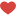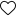Hi Data science enthusiasts,

Hope you are doing well !!!!

FLAML – Fast and Lightweight AutoML primarily developed by the team of Microsoft research. FLAML is one more contender In the open source Automl field( Pycaret,Evalml,H2O are already there).  Main USP of this framework is that it selects all the learners and hyperparameters by itself. User don’t have to provide a list of parameters to run the model. FLAML favors the simple models at first and later penalizes it if the error is not getting reduced.

Unique thing about this framework is you can specify the time limit for how long the model should work and FLAML will try to find out all the possible algorithms and hyperparameters for that particular problem

We are going to run a classification task using FLAML.

Data we are going to use is Pima Indians Diabetes Dataset which has target variable as class whether a person will be having diabetes or not? There are 768 observations with 8 input variables and 1 output variable

The variable names are as follows:

1. Number of times pregnant.
2. Plasma glucose concentration a 2 hours in an oral glucose tolerance test.
3. Diastolic blood pressure (mm Hg).
4. Triceps skinfold thickness (mm).
5. 2-Hour serum insulin (mu U/ml).
6. Body mass index (weight in kg/(height in m)^2).
7. Diabetes pedigree function.
8. Age (years).
9. Class variable (0 or 1).

How to install :-

`pip install flaml`

Lets import all the required libraries

```import pandas as pd import numpy as np ```

`df = pd.read_csv("https://raw.githubusercontent.com/jbrownlee/Datasets/master/pima-indians-diabetes.csv",header = None)`

Data does not have column names hence we will enter the names manually

```df.columns = ["Number of times pregnant","Plasma glucose","Diastolic blood pressure","Triceps skin fold thickness","2-Hour serum insulin","bmi","Diabetes pedigree function","Age","Class"] df.columns```

Lets check the shape of the data which is  768 rows and 9 columns

`df.shape`

Check if the data is having any missing values or not?

`df.isnull().sum()`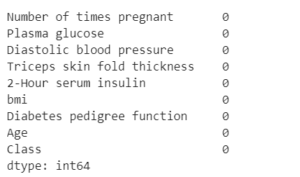looks like we don’t have any missing values (thank god)

Print the head of the data frame to have a glimpse of the data

`df.head()`
``` import seaborn as sns sns_plot =sns.countplot(df['Class']) fig = sns_plot.get_figure() fig.savefig("Class imbalance.png")```From the above image we can see our data is imbalanced hence, we will try to maximize the F1 score instead of Accuracy to get the actual accuracy of the model

lets divide the data in X and y for the train test split, we will do a 80:20 split on the data as we don’t have higher amount of training data.

```X = df.drop(['Class'], axis = 1) y = df['Class'] from sklearn.model_selection import train_test_split X_train, X_test, y_train, y_test = train_test_split(X, y, test_size=0.2, random_state=27)```

Now,import the FLAML Automl framework

```from flaml import AutoML automl = AutoML()```

lets configure all the settings , we need to maximize the actual accuracy hence we will try to maximize F1 score

```settings = { "time_budget": 300,  # total running time in seconds "metric": 'f1',  # primary metrics can be chosen from: ['accuracy','roc_auc','f1','log_loss','mae','mse','r2'] "task": 'classification',  # task type  classification or regression "log_file_name": 'diabetes_experiment.log',  # flaml log file }```

Run the automl code, code will run for 300 seconds or until it converges.

`automl.fit(X_train=X_train, y_train=y_train, **settings)`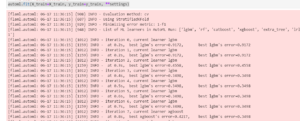Once the model is run , you will get an output like this:-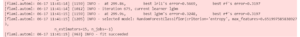Lets try to find out the best model and hyperparameters for the model

```print('Best ML leaner:', automl.best_estimator) print('Best hyperparmeter config:', automl.best_config) print('Best accuracy on validation data: {0:.4g}'.format(1-automl.best_loss)) print('Training duration of best run: {0:.4g} s'.format(automl.best_config_train_time))```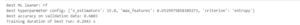Check which is the model selected by FLAML

`automl.model`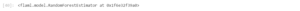After completing all the iterations Random forest comes out as the best model and its hyperparameters are also provided.

we can save the model using pickle by

```import pickle with open('automl.pkl', 'wb') as f: pickle.dump(automl, f, pickle.HIGHEST_PROTOCOL)```

Lets run the prediction on the validation set
``` y_pred = automl.predict(X_test) print('Predicted labels', y_pred) print('True labels', y_test) y_pred_proba = automl.predict_proba(X_test)[:,1]```

Check all the performance metrics
``` from flaml.ml import sklearn_metric_loss_score print('accuracy', '=', 1 - sklearn_metric_loss_score('accuracy', y_pred, y_test)) print('roc_auc', '=', 1 - sklearn_metric_loss_score('roc_auc', y_pred_proba, y_test)) print('log_loss', '=', sklearn_metric_loss_score('log_loss', y_pred_proba, y_test)) print('f1', '=', 1 - sklearn_metric_loss_score('f1', y_pred, y_test))```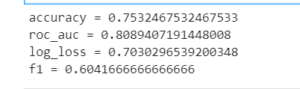from the results we can see we have got F1 score as 60 and accuracy as 75%  which is not bad as we have not performed any feature engineering or data preprocessing.

How to get logs of the model ?

```from flaml.data import get_output_from_log time_history, best_valid_loss_history, valid_loss_history, config_history, train_loss_history = \ get_output_from_log(filename=settings['log_file_name'], time_budget=60)```
``` for config in config_history: print(config)```

To check the model learning curve we can use

```import matplotlib.pyplot as plt import numpy as np plt.title('Learning Curve') plt.xlabel('Wall Clock Time (s)') plt.ylabel('Validation Accuracy') plt.scatter(time_history, 1 - np.array(valid_loss_history)) plt.step(time_history, 1 - np.array(best_valid_loss_history), where='post') plt.show()```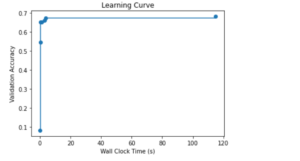From the plot we can see model did reached it peak around 120 seconds time.

This is how we can use Automl Framework to do difficult task of finding the best hyperparameters with some few simple line of codes.

Apart from Datarobot which is a subscription based tool, I personally think automl has a long way to go when it comes to corporates putting Automl models into production but FLAML can surely help data scientist’s to find the best hyperparameters.

Thanks and regards,

Hunaidkhan Pathan

You can find the complete code in my Github Repository.

References ,

https://github.com/microsoft/FLAML

https://machinelearningmastery.com/standard-machine-learning-datasets/